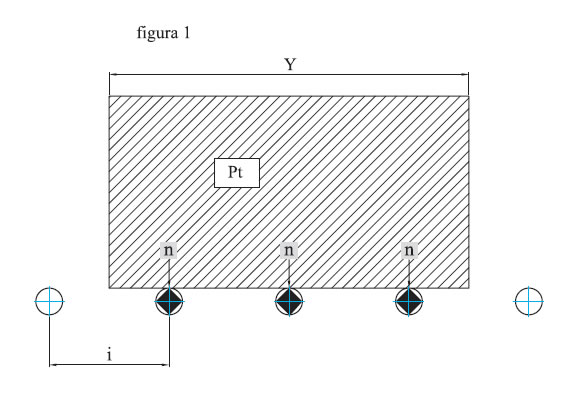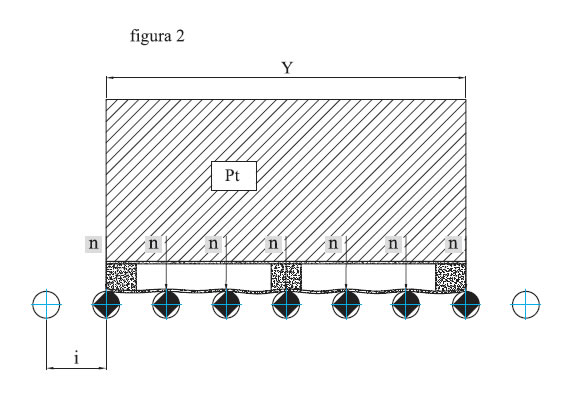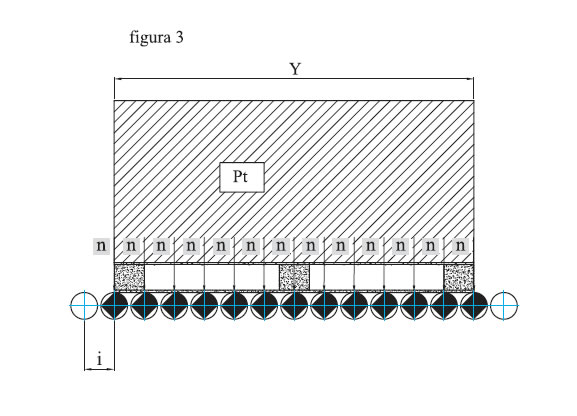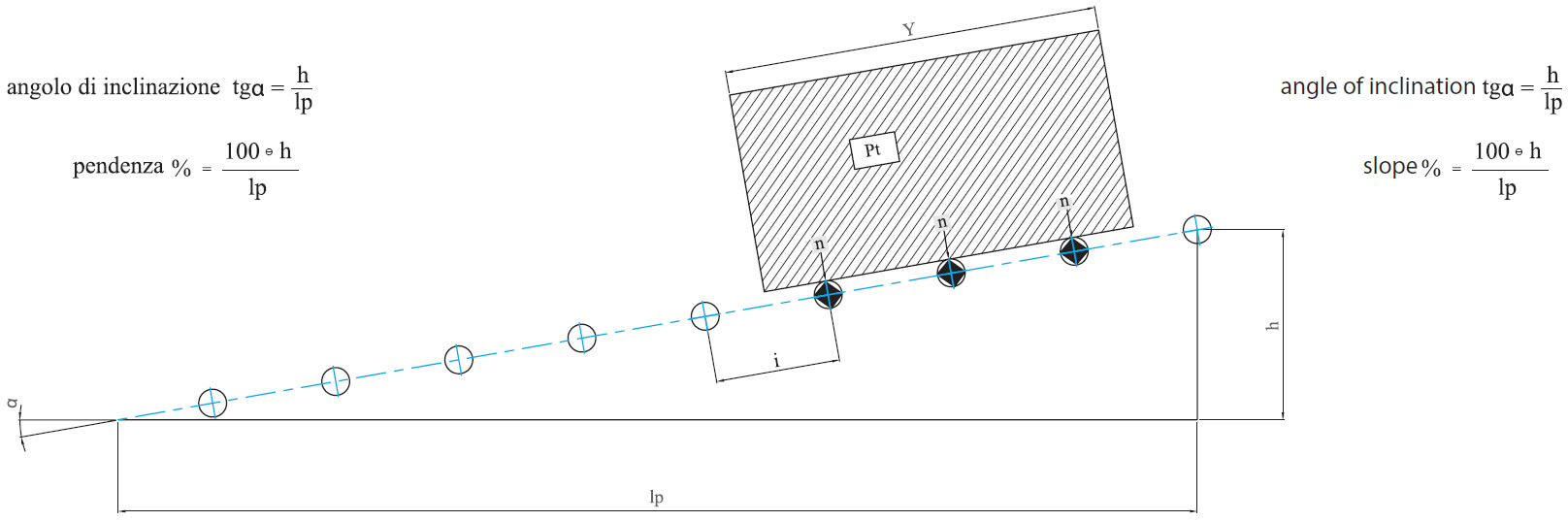# Technical details### Description of a roller conveyor

It is composed of a frame that supports a series of rollers.
The load can be moved by the force of gravity or by a motorized unit. The frames can be constructed in straight lines or be curved.
The load to convey must have a smooth surface, be solid and long and must rest on at least 3 rollers (fig. 1).
The distance between the roller centrelines must be reduced when the support surfaces so require (figures 2-3).

y = length of the load
i = distance between the roller centrelines
n = number of rollers subjected to the load
pt = weight of the load

example
i = y/n

### Distribution of the load on the rollers

pt = weight of the load
p = nominal load bearing on one roller (daN)
pm = maximum load bearing on one roller (daN)
n = number of the rollers subjected to the load
W = coefficient of support of the conveyed load
W = 1.5 when the number of rollers subjected to the
load is n≥3 and the support surface is not extremely
rigid
W = 2 when the number of rollers subjected to the load is n > 3 and the support surface is extremely rigid.
The formula indicates the maximum load for determining the choice of roller.### Slope

The calculation of the percentage slope required for moving the load correctly is constrained by a coefficient of friction that is difficult to evaluate as it is necessary to take account of the friction between the load and the rollers, the friction of the bearings, the ratio between the weight of the rollers and the load, and countless incidental causes such as, for example, rubbing by the material of the conveyed load up against the containment guides.
If the loads are heavy, they cannot be conveyed for great distances unless decelerating rollers are employed.
Because it is impossible to determine the slope required for conveying the load with certainty, we recommend carrying out a practical test and, for indicative purposes only, we propose the following maximum values.

2% for metal loads
4% for wooden loads
8% for cardboard loads

α=angle of inclination
lp=plan length of the conveyor
h=height drop of the conveyor